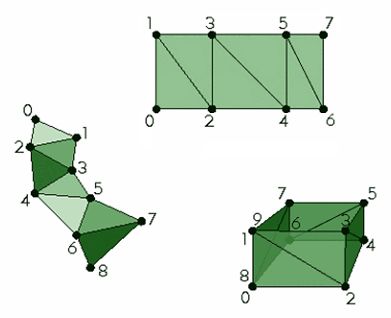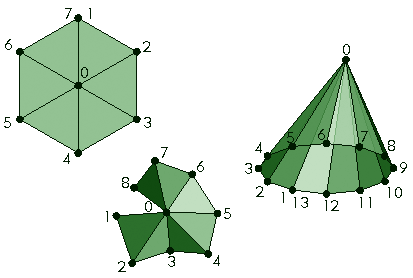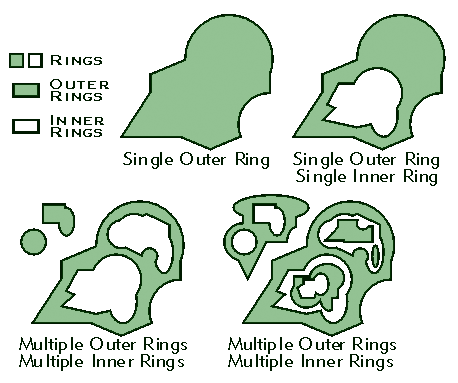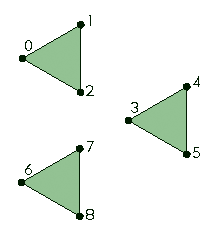﻿ esriPatchType Enumeration—ArcGIS Pro
ArcGIS Pro 2.6 API Reference Guide
esriPatchType Enumeration

 ArcGIS.Core.Geometry Namespace : esriPatchType Enumeration
Describes the type of the patch.
Syntax
`public enum esriPatchType : System.Enum, System.IComparable, System.IConvertible, System.IFormattable  `
```Public Enum esriPatchType
Inherits System.Enum
Implements System.IComparable, System.IConvertible, System.IFormattable ```
Members
MemberDescription
FirstRing The first ring created when constructing the polygon.
Ring A ring of the same polygon as the previous patch.
TriangleFan A continuous fan of 3-dimensional triangles such that the first point defines the origin.
Triangles A collection of 3-dimensional triangles.
TriangleStrip A continuous linked strip of 3-dimensional triangles.
Remarks

Each part in a multipatch is called a patch. A patch can be a triangle strip, triangle fan, a group of triangles, or a ring. If the patch is a ring, it can be either the first ring of a polygon or another ring in the same polygon. The first ring means that it was the first ring created for the polygon when the multipatch was constructed. It doesn't necessarily mean that it is an outer ring and subsequent rings in the polygon are inner rings.

A triangle strip is a continuous linked strip of 3-dimensional triangles such that every vertex after the first two completes a new triangle. A new triangle is always formed by connecting the new vertex with its two immediate predecessors. For example, a triangle strip with six points has triangles defined by the point indices (0, 1, 2), (2, 1, 3), (2, 3, 4), (4, 3, 5).A triangle fan is a continuous fan of 3-dimensional triangles such that the first point defines the origin. All triangles in the fan share the origin as a common pivot point. Every vertex after the first two completes a triangle, and a new triangle is formed by connecting the new vertex to its immediate predecessor and the origin. For example, a triangle fan with six points has triangles defined by point indices (0, 1, 2), (0, 2, 3), (0, 3, 4), (0, 4, 5).A ring is a geometric element from which polygons are constructed, defined by an area bounded by one closed sequence of connected segments. If the type of a patch is esriPatchType.FirstRing, then it was the first ring created when constructing the polygon. If the type of the patch is esriPatchType.Ring, then it is part of the same polygon as the previous patch.Triangles is a collection of 3-dimensional triangles such that each consecutive triplet of vertices defines a new triangle. The number of vertices in patch of type esriPatchType.Triangles must be multiple of three. For example, a patch of type esriPatchType.Triangles with nine points has triangles defined by point indices (0, 1, 2), (3, 4, 5), (6, 7, 8).Inheritance Hierarchy

System.Object
System.ValueType
System.Enum
ArcGIS.Core.Geometry.esriPatchType

Requirements

Target Platforms: Windows 10, Windows 8.1, Windows 7